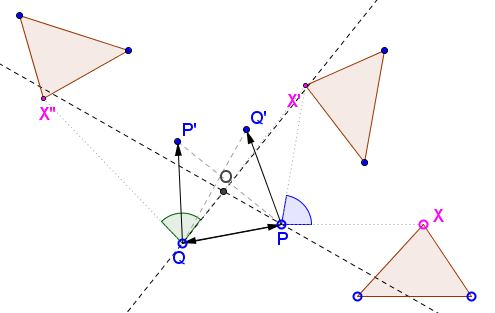# Product of Rotations

The product, or the sum as its often called, of two rotations is either a rotation or a translation, both of which can be trivial, i.e., move nothing. Depending on the context, one may treat the product of two rotations which is trivial as either a rotation or a translation.

The product of two rotations through angles $\alpha$ and $\beta$ is a rotation through angle $\alpha + \beta.$ Its center, which exists if the product is not trivial, can be found constructively.Let there be given two rotations $g_{P}$ and $g_{Q}$ with centers $P$ and $Q.$ Assume $g_{P}$ is executed first, $g_{Q}$ second. $g_{P}$ leaves $P$ in place. Denote $g_{Q}(P) = P'.$ $g_{P}$ carries some point $Q'$ onto $Q$ which is then left in place by $g_{Q}.$ The point $O$ that lies at the intersection of perpendicular bisectors of $QQ'$ and $PP'$ is equidistant from $P$ and $P'$ and also from $Q$ and $Q'.$

Now prove that $\angle POP' = \angle Q'OQ = \angle PQP' + \angle Q'PQ.$ Finally, consider what happens with an arbitrary point (or two) under two successive rotations.

(The fact just established provides a powerful tool for solving geometric problems, see, for example, proofs of Bottema's theorem and an analogue.)### Geometric Transformations